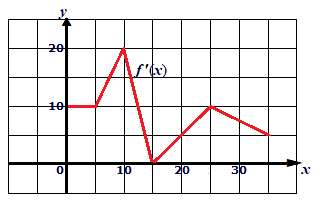# Use the graph of f'(x), given above, and the fact that f(0) = 40 to compute the following values....

## Question:Use the graph of {eq}f'(x) {/eq}, given above, and the fact that {eq}f(0) = 40 {/eq} to compute the following values.

a. {eq}f(5) {/eq}

b. {eq}f(15) {/eq}

## Integration

When we are f'(x) and asked to find the values of f(x) the best method is to integrate the f'(x).

{eq}\int f'(x) = f(x) +C {/eq}

To find the constant use the known values of the function.

## Answer and Explanation:

Given:

f(0) = 40.

Now initially till x=5;

f'(x) = 10

Now integrating we get:

{eq}\int f'(x) = \int 10 = 10x+ C \\ f(x) = 10x+C \\ f(0) = 40= C \\ f(x) = 10x+40 \\ f(5) = 90. {/eq}

Now for 10>x>5 we can see that f'(x) is of the form:

{eq}f'(x) = 2x \\ \int f'(x) = \int 2x = x^2+C \\ f(x) = x^2+C \\ f(5) = 90= 25+C \\ C = 65 \\ f(x) = x^2+65 \\ f(10) = 165. {/eq}

Now for 10<x<15:

{eq}f'(x) = 60-4x \\ \int f'(x) = \int 60-4x = 60x-2x^2 \\ f(x) = 60x-2x^2+C \\ f(10) = 165 = 600-200+C \\ C = -235 \\ f(15) = 60*15-2*225-235 = 215. {/eq}

#### Learn more about this topic:Graphs: Types, Examples & Functions

from High School Algebra II: Help and Review

Chapter 16 / Lesson 11
353K